# SINUMERIK系统参数编程

R0=...

R249=...

##### 3.说明

R0...R99 - 可以自由使用

R100...R249 -加工循环传递参数

##### 4.赋值

R0=3.5678 R1=-37.3 R2=2 R3=-7 R4=-45678.1234

（10－300...10＋300）.

EX值范围：－300到＋300

R0=-0.1EX-5 ；意义：R0=-0.000 0001

R1=1.874EX8 ；意义：R1=187 400 000

##### 5.给其他的地址赋值

N10 G0 X=R2 ；给X轴赋值

##### 1.功能

1)标记符用于标记程序中所跳转的目标程序段，用跳转功能可以实现程序运行分支。

2)标记符可以自由选取，但必须由2一个字母或数字组成，其中开始两个符号必须是字母或下划线。

3)跳转目标程序段中标记符后面必须为冒号。标记符位于程序段段首。如果程序段有段号，则标记符紧跟着段号。

4)在一个程序段中，标记符不能含有其它意义。

##### 2.程序举例

N10 MARKE1:G1 X20 ；MARKE1为标记符，跳转目标程序段 ...

TR789:G0 X10 Z20 ；TR789为标记符，跳转目标程序段没有段号

##### 1.功能

NC程序在运行时以写入时的顺序执行程序段。

##### 2.编程

GOTOF Lable ；先前跳转

GOTOB Lable ；向后跳转

AWL 说明

GOTOF 向前跳转（向程序结束的方向跳转）

GOTOB 向后跳转（向程序开始的方向跳转）

Lable 所选的标记符

##### 2.编程

IF条件GOTOF Lable ；先前跳转

IF条件GOTOB Lable ；向后跳转

 AWL 说明 GOTOF 向前跳转(向程序结束的方向跳转) GOTOB 向后跳转(向程序开始的方向跳转) Lable 所选的标记符 IF 跳转条件导入符 条件 作为条件的计算参数,计算表达式
##### 3.比较运算
 运算符 意义 = = 等于 ＜＞ 不等 ＞ 大于 ＜ 小于 ＞ = 大于或等于 ＜ = 小于或等于

##### 4.比较运算编程举例

R1＞1 ；R1大于1

1＜R1 ；1小于R1

R1＜R2+R3 ；R1小于R2加R3

R6＞=SIN(R7*R7) ；R6大于或等于SIN(R7)2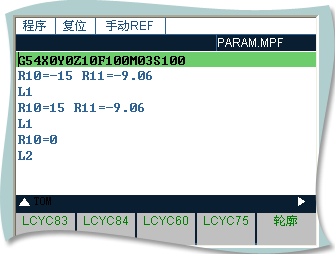L1(半球):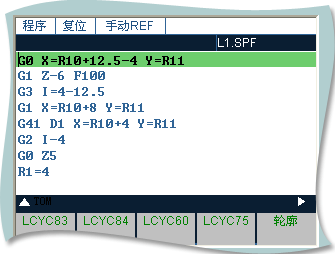L2(半球):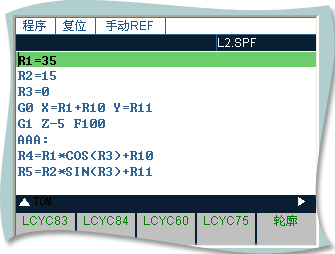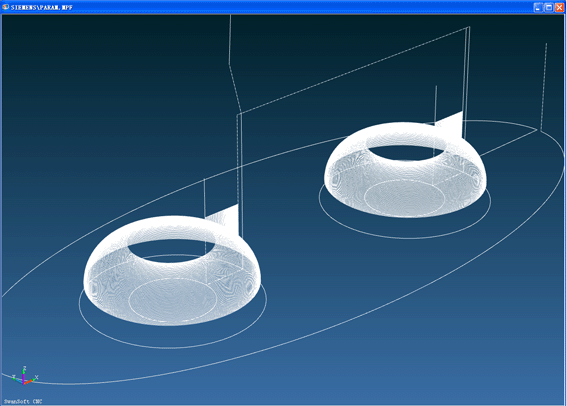G54X0Y0Z10F100M03S100

R10=-15 R11=-9.06

L1

R10=15 R11=-9.06

L1

R10=0

L2

G0 X=R10+12.5-4 Y=R11

G1 Z-6 F100

G3 I=4-12.5

G1 X=R10+8 Y=R11

G41 D1 X=R10+4 Y=R11

G2 I-4

G0 Z5

R1=4

R2=90

AAA:

R3=R1*COS(R2)+4+R10

R4=R1*SIN(R2)-R1

G0 X=R3 Y=R11

G1 Z=R4 F300

G2 I=R10-R3

G0 Z1

R2=R2-1

IF R2>=0 GOTOB AAA

G0 Z10

M17

R1=35

R2=15

R3=0

G0 X=R1+R10 Y=R11

G1 Z-5 F100

AAA:

R4=R1*COS(R3)+R10

R5=R2*SIN(R3)+R11

G1 X=R4 Y=R5 F100

R3=R3+1

IF R3<=360 GOTOB AAA

G0 Z5

M17

##### 3.程序结束

RET要求占用一个独立的程序段。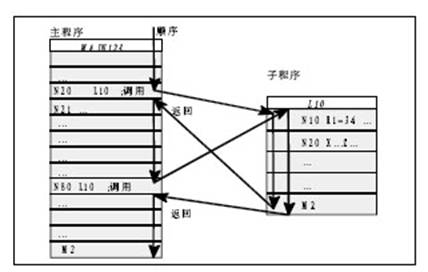－ 开始两个符号必须时字母

－ 其它符号为字母，数字或下划线

－ 最多8个字符

－ 没有分隔符

##### 5.子程序调用

N10 L785 P3 ；调用子程序L785

N20 WELLE7 ；调用子程序WELLE7

##### 6.程序重复调用

N10 L785 P3 ；调用子程序L785 ,运行3次